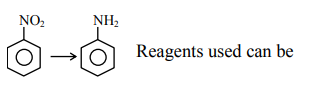# The total number of reagents from those given below, that can convert nitrobenzene into aniline is - (Integer answer)Question:

The total number of reagents from those given below, that can convert nitrobenzene into aniline is - (Integer answer)

I. $\mathrm{Sn}-\mathrm{HCl}$

II. $\mathrm{Sn}-\mathrm{NH}_{4} \mathrm{OH}$

III. $\mathrm{Fe}-\mathrm{HCl}$

IV.Zn - HCl

V. $\mathrm{H}_{2}-\mathrm{Pd}$

VI. $\mathrm{H}_{2}$ - Raney Nickel

Solution:(i) $\mathrm{Sn}+\mathrm{HCl}$

(ii) $\mathrm{Fe}+\mathrm{HCl}$

(iii) $\mathrm{Zn}+\mathrm{HCl}$

(iv) $\mathrm{H}_{2}-\mathrm{Pd}$

(v) $\mathrm{H}_{2}$ (Raney $\mathrm{Ni}$ )